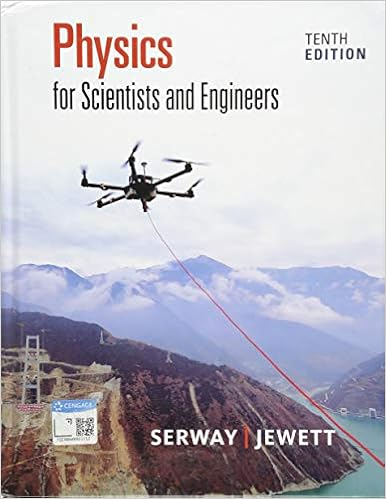Momentum and Collision Simulation Lab _0.pdf - Momentum...

• Lab Report
• 2

This preview shows page 1 - 2 out of 2 pages.

We have textbook solutions for you!
The document you are viewing contains questions related to this textbook.The document you are viewing contains questions related to this textbook.
Chapter 11 / Exercise 49
Physics for Scientists and Engineers
Jewett/SerwayExpert Verified
Momentum Internet Lab Momentum and Collisions Name: Date: Period: Website: Play with the Sims Physics Motion Collision Lab Introduction: When objects move, they have momentum . Momentum, p, is the product of an object’s mass (kg) and its velocity (m/s). The unit for momentum, p, is kg·m/s. During a collision objects transfer momentum to each other, resulting in different motions than before the collision. In this activity you will study the motion colliding objects. momentum = m × v ELASTIC Collisions 1 1before 2 2before 1 1after 2 2after m v + m v = m v + m v 1. What defines a collision as being elastic? 2. Simulate the four elastic collisions below. Complete the table using math formulas and the simulation. BEFORE COLLISION p total AFTER COLLISION # m 1 m 2 v 1 v 2 v 1 v 1 2.0 kg 2.0 kg 1.5 m/s 0 kg·m/s 2 2.5 kg 5.0 kg -1.0 m/s 0 kg·m/s 3 3.0 kg 6.0 kg 2.0 m/s 0.0 m/s 4 6.0 kg 2.0 m/s -1.0 m/s 8.0 kg·m/s 3. Two objects with the same mass move toward each other with the same speed and experience an elastic collision. 2
We have textbook solutions for you!
The document you are viewing contains questions related to this textbook.The document you are viewing contains questions related to this textbook.
Chapter 11 / Exercise 49
Physics for Scientists and Engineers
Jewett/SerwayExpert Verified
•••